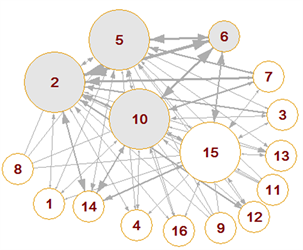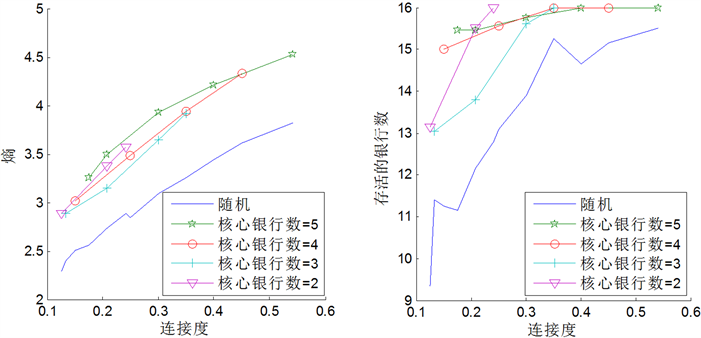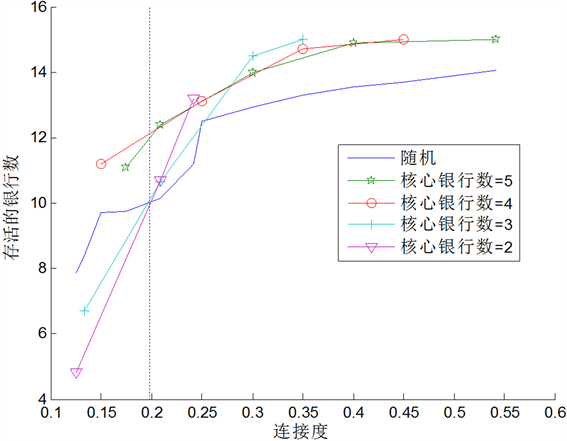# 核心边缘网络结构对银行系统稳定性影响研究The CP Network Structure and Systemic Risk in Interbank Market

DOI: 10.12677/AAM.2017.69132, PDF, HTML, XML, 下载: 889  浏览: 1,106  国家自然科学基金支持

Abstract: The network topology structure of the interbank network has an important effect on the stability of bank system; most of the current researches study the effect of the random network on the stability of bank system. However many empirical investigations find that the core-periphery (CP) is an important network characteristic of the interbank market, therefore, the present paper constructs the CP network structure of the interbank market, and then constructs a dynamical evolutional bank network system model, furthermore studies the effect of CP network structure on the stability of the bank system. The research results show that when the periphery banks suffer from the deposits shocks, the CP network is more stable than the random network. However, when the core banks face the deposits shocks, there are three cases according to the connectivity of the banking system. In the case of the lower connectivity and small number of core banks, then the random network is more stable; in the case of the lower connectivity and big number of core banks, or in the case of the bigger connectivity, the CP network is more stable.

1. 引言

2007年，美国爆发次贷危机，多家大型的投资银行及其他金融机构相继倒闭，致使全球主要金融市场出现流动性危机，给全球的银行系统和金融系统造成了巨大的冲击，最终引发了一场全球性金融危机。此次金融危机表现出了全球一体化后银行系统的脆弱性和关联性。银行间日趋紧密的联系似乎有助于降低金融危机爆发的可能性，然而一旦发生了，这个危机就会演变成全球性的危机。因此，研究银行系统性风险已成为国内外学术界和政府监管机构关注的焦点。这一研究无疑对防范和化解系统性风险、维护金融稳定具有重要意义。

2000年，Allen和Gale  ，Freixas  等人的研究最早提出：银行系统的稳定性与银行间拆借网络结构有关。此后，学者们对不同国家的银行间拆借网络进行实证研究，发现真实的银行拆借网络与其他领域的网络如国家电网、社区网络等同样具有复杂网络的结构特征。他们利用银行间风险暴露的相关数据，发现银行网络结构具有小世界、无标度等复杂网络结构特征      。并且国内外均有学者通过仿真或实证的方式分析了无标度网络对银行间市场的影响  -  。然而，许多无标度网络中主张的统计学理论依据受到了质疑  ，其原因应归结为无标度网络的渐进性。无标度网络中，即使在数据集很大的情况下也只能观测到极少量的极端度值。现实中，银行系统内的节点往往都是有限的并且规模是固定的，所以把银行系统构建成无标度网络(持续增长)并不合适  。Craig和von Peter  认为银行间拆借市场是有层次的，大多数的银行不是直接发生借贷而是通过货币中心银行作为中介，因此建立“货币中心系统”模型更为合适。Craig和von Peter建立的核心边缘模型首次将“货币中心银行”等概念进行了定量的描述，研究发现德国的银行网络中核心银行占2.7%。随后，in’t Veld和van Lelyveld  同样发现核心边缘网络模型能最好地描绘荷兰银行同业市场的网络结构。

2. 模型

2.1. 核心边缘网络结构构建

a) 核心银行之间全连接

${J}_{ij}=1,\text{\hspace{0.17em}}\left(\forall i\in H,\forall j\in H且i\ne j\right)$ ;

b) 边缘银行之间无连接

${J}_{ij}=0,\text{\hspace{0.17em}}\left(\forall i\in P,\forall j\in P\right)$ ;

c) 核心银行至少与边缘银行存在一借一贷的连接(随机连接，连接个数取决于银行系统的连接度)

${J}_{ij}=1,\text{\hspace{0.17em}}\left(\exists i\in H,\exists j\in P\right)$ .

$J=\left(\begin{array}{cc}{m}_{1}& {m}_{2}\\ {m}_{3}& {m}_{4}\end{array}\right)=\begin{array}{c}\begin{array}{l}\\ \\ H\\ \end{array}\\ \begin{array}{l}\\ \\ P\\ \end{array}\end{array}\begin{array}{c} H P \\ \left(\begin{array}{cccccccc}0& 1& \cdots & 1& \text{|}& 1& \cdots & 0\\ 1& \ddots & \ddots & ⋮& \text{|}& 1& \cdots & 0\\ ⋮& \ddots & \ddots & 1& \text{|}& ⋮& \ddots & ⋮\\ 1& \cdots & 1& 0& \text{|}& 1& \cdots & 0\\ -& -& -& -& \text{|}& -& -& -\\ 1& 1& \cdots & 1& \text{|}& & & \\ ⋮& ⋮& \ddots & ⋮& \text{|}& & 0& \\ 0& 0& \cdots & 0& |& & & \end{array}\right)\end{array}$ (1)

2.2. 动态银行网络系统模型

$IA+I+L=IL+A+V$ (2)

$L=A+V-I+\left(IL-IA\right)$ (3)

${L}_{t}^{k}={A}_{t-1}^{k}+{V}_{t-1}^{k}-{\sum }_{s=1}^{\tau }{I}_{t-s}^{k}$ (4)

${\stackrel{^}{L}}_{t}^{k}={L}_{t}^{k}+\left({A}_{t}^{k}-{A}_{t-1}^{k}\right)-{r}_{a}{A}_{t-1}^{k}+\rho {\sum }_{s=1}^{\tau }{I}_{t-s}^{k}+{I}_{t-\tau }^{k}+{r}_{b}\left(I{A}_{t-1}^{k}-I{L}_{t-1}^{k}\right)$ (5)

${A}_{t}^{k}=|{\stackrel{¯}{A}}_{k}+{\stackrel{¯}{A}}_{k}{\sigma }_{A}{\epsilon }_{t}|,\text{\hspace{0.17em}}{\epsilon }_{t}\in N\left(0,1\right)$ (6)

${\stackrel{˜}{L}}_{t}^{k}={\stackrel{^}{L}}_{t}^{k}-{D}_{t}^{k}-{I}_{t}^{k}$ (7)

${D}_{t}^{k}=\mathrm{max}\left\{0,\mathrm{min}\left\{\rho {\sum }_{s=1}^{\tau }{I}_{t-s}^{k}-{r}_{a}{A}_{t-1}^{k},{\stackrel{^}{L}}_{t}^{k}-\beta {A}_{t}^{k},{\stackrel{^}{L}}_{t}^{k}+{\sum }_{s=1}^{\tau -1}{I}_{t-s}^{k}-\left(1+\chi \right){A}_{t}^{k}\right\}\right\}$ (8)

${I}_{t}^{k}=\mathrm{min}\left\{\mathrm{max}\left\{0,{\stackrel{^}{L}}_{t}^{k}-{D}_{t}^{k}-\beta {A}_{t}^{k}\right\},{\omega }_{t}^{k}\right\}$ (9)

${\omega }_{t}^{k}=|{\stackrel{¯}{\omega }}_{k}+{\stackrel{¯}{\omega }}_{k}{\sigma }_{\omega }{\eta }_{t}|,\text{\hspace{0.17em}}{\eta }_{t}\in N\left(0,1\right)$ (10)

${A}_{t}^{k}=|{\stackrel{¯}{A}}_{k}+{\stackrel{¯}{A}}_{k}p{\sigma }_{A}{\epsilon }_{t}|,\text{\hspace{0.17em}}{\epsilon }_{t}\in N\left(0,1\right)$ (11)

3. 仿真与结论

3.1. 核心边缘网络结构估算

3.2. 边缘银行受到存款冲击的情况

 Math_69#

(a) (b)(a)(b)

Figure 2. Interbank lending algorithmTable 2. List of core banks and periphery banks in four cases(a) (b)

Figure 3. The stability of bank system when the periphery banks suffered deposits shocksFigure 4. The stability of bank system when the core banks suffered deposits shocks

3.3. 核心银行受到存款冲击的情况

4. 结论

1) 在边缘银行受到存款冲击的情形下，核心边缘网络的银行系统稳定性高于随机网络的银行系统。拆借资金在银行间的分布越均匀，即银行的平均风险越低越有利于分散风险，越利于提高银行系统的稳定性，系统越稳定。此种情况下监管部门应当防止银行之间过度集中的拆借交易行为。

2) 在核心银行受到存款冲击的情形下，当连接度小时，若核心银行总数少，随机网络更稳定，且拆借资金在银行间的分布越集中系统越稳定。此种情况下监管部门应视具体情况决定是否提供外部救助，如果拆借频繁的银行是受到冲击的银行时应选择救助，如果拆借频繁的银行不是受到冲击的银行时选择让银行间互助。但当核心银行总数大或连接度大时，核心边缘网络更稳定，且拆借资金在银行间的分布越均匀系统越稳定。

  陈国进, 马长峰. 金融危机传染的网络理论研究述评[J]. 经济学动态, 2010(2): 116 -120.  Allen, F. and Gale, D. (2000) Financial Contagion. Journal of Political Economy, 108, 1-33. https://doi.org/10.1086/262109  Freixas, X., Parigi, B. and Rochet, J.C. (2000) Systemic Risk, Interbank Relations and Liquidity Provision by the Central Bank. Journal of Money, Credit and Banking, 32, 611-640. https://doi.org/10.2307/2601198  Boss, M., Elsinger, H. and Summer, M. (2004) The Network Topology of the Interbank Market. Quantitative Finance, 4, 677-684. https://doi.org/10.1080/14697680400020325  Gabrieli, S. (2011) The Microstructure of the Money Market before and after the Financial Crisis: A Network Perspective. CEIS Tor Vergata Research Paper Series, 9, No. 1.  Iori, G., Demasi, G., Precup, O.V., et al. (2008) A Network Analysis of the Italian Overnight Money Market. Journal of Economic Dynamics & Control, 32, 259-278. https://doi.org/10.1016/j.jedc.2007.01.032  Craig, B. and Von Peter, G. (2014) Interbank Tiering and Money Center Banks. Journal of Financial Intermediation, 23, 322-347. https://doi.org/10.1016/j.jfi.2014.02.003  Degryse, H. and Nguyen, G. (2007) Interbank Exposures: An Empirical Examination of Contagion Risk in the Belgian Banking System. International Journal of Central Banking, 3, 123-171.  高国华, 潘英丽. 基于资产负债表关联的银行系统性风险研究[J]. 管理工程学报, 2012, 26(4): 162-168.  邓晶, 曹诗男, 潘焕学, 秦涛. 基于银行间市场网络的系统性风险传染研究[J]. 复杂系统与复杂性科学, 2013, 10(4): 76-85.  隋聪, 迟国泰, 王宗尧. 网络结构与银行系统性风险[J]. 管理科学学报, 2014, 17(4): 57-70.  蔡世民, 洪磊, 傅忠谦, 周佩玲. 基于复杂网络的金融市场网络结构实证研究[J]. 复杂系统与复杂性科学, 2011, 8(3): 29-33.  Krause, A. and Giansante, S. (2012) Interbank Lending and the Spread of Bank Failures: A Network Model of Systemic Risk. Journal of Economic Behavior and Organization, 83, 583-608. https://doi.org/10.1016/j.jebo.2012.05.015  隋聪, 王宗尧. 银行间网络的无标度特征[J]. 管理科学学报, 2015, 18(12): 18-26.  Stumpf, P.H. and Porter, M.A. (2012) Critical Truths about Power Laws. Science, 335, 665-666. https://doi.org/10.1126/science.1216142  in’t Veld, D. and van Lelyveld, I. (2014) Finding the Core: Network Structure in Interbank Markets. Journal of Banking & Finance, 49, 27-40. https://doi.org/10.1016/j.jbankfin.2014.08.006  Iori, G. (2006) Systemic Risk on the Interbank Market. Journal of Economic Behavior & Organization, 61, 525-542. https://doi.org/10.1016/j.jebo.2004.07.018